The SSM Procedure

Multivariate ARMA

You can specify a state vector that follows a multivariate autoregressive, moving average (VARMA) model by using the STATE statement option TYPE=VARMA . The autoregressive and moving average orders can be either 0 or 1 (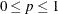and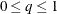)—that is, only VAR(1), MA(1), and VARMA(1,1) models can be specified. The notation and the state space form of the VARMA model described here is taken from Reinsel (1997), which is a good reference for VARMA modeling.

A dim-dimensional vector process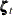follows a zero-mean, autoregressive order p, moving average order q (VARMA(p, q)) model if it satisfies the following matrix difference equation: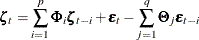Here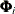and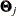are dim-dimensional square matrices and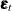is a dim-dimensional, Gaussian, white noise sequence with covariance matrix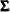. If autoregressive order p is 0, the term that involvesis absent; similarly, if the moving average order q is 0, the term that involvesis absent. Since AR and MA orders can be at most 1, the subscripts ofandcan be ignored in this discussion—when applicable, an AR coefficient matrix is denoted by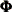and an MA coefficient matrix is denoted by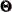. The unknown elements of,, andconstitute the parameter vector that is associated with a VARMA state. The processdefined by the VARMA difference equation is stationary and invertible (Reinsel, 1997) if and only if the eigenvalues ofandare strictly less than 1 in magnitude. By default, the SSM procedure imposes these stationarity and invertibility restrictions onand. However, you can specifyto be an identity matrix, in which case the resulting process is nonstationary.

A VARMA model can be cast into a state space form. The state space form used by the SSM procedure is described in Reinsel (1997, pp 52–53). The system matrices for the supported VARMA models are as follows:

• The VAR(1) form is the simplest. In this case, the underlying state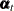is the same as the VAR(1) process. Therefore,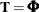and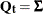.

• Takingequal to the zero matrix if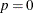, the VARMA(1,1) and MA(1) cases can be treated together. In this case, the underlying stateis 2*dim dimensional and the desired VARMA processcorresponds to its first dim elements. Let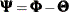. Then, in the blocked form,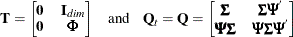Unlessis restricted to be identity, the underlying stateis stationary and the covariance of the initial condition is computed by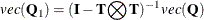where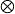denotes the Kronecker product and theoperation on a matrix creates a vector formed by vertically stacking the rows of that matrix. Ifis restricted to be identity, the initial condition is fully diffuse.